# Convert Date to text with Text Function in Excel

This post will guide you how to convert dates to text string using Text Function in Microsoft Excel.

The Excel TEXT function converts a numeric value into text string with a specified format. You can convert all the standard number formats such as: dates, times, currency to Text string in excel. The Date and Time are recognized as numeric values in Excel. So you can use these dates and time values in calculation. Such as, you can perform addition operation for those Dates values.

When you want the dates and Time values as text string, you can use TEXT function in excel to convert dates to text in a specific format code. For example, The TEXT function can use the following patterns, like as: “mm/dd/yyyy”, “yyyy/mm/dd”, etc.

The syntax of the TEXT function is as below:

`= TEXT (value, Format code)`

## Convert Date to Text with Text Function

If you want to convert a specified Date value to Text string, just refer to the below examples:

1# Assuming that convert the date value “12/11/2018” to “11-Dec-2018”, you can use the format code as: “dd-mmm-yyyy”, then used it in the Text function as follows:

`=TEXT(B1,"dd-mmm-yyyy")`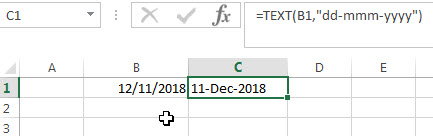2# If you want to change the date “12/11/2018” to “11-12-2018”, you can use the format code as: “dd-mm-yyyy”, then used it in the following Text function in excel:

=Text(B1,”dd-mm-yyyy”)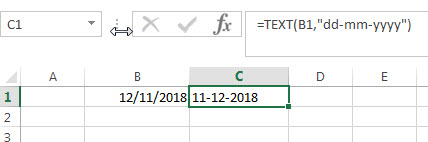3# If you want to convert the date “12/11/2018” to “Dec-11-2018”, you can use the format code as: “mmm-dd-yyyy” in the following Text function:

`=TEXT(B1,"mmm-dd-yyyy")`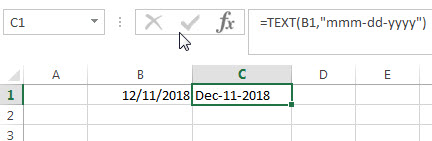## Convert Current Date to Text

If you want to convert the current date into text string, then you can use the TODAY function within the TEXT function in excel, let’s see the following TEXT formula:

`=TEXT(TODAY(),"dd/mm/yyyy")`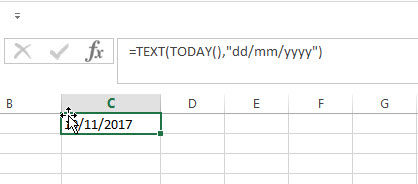## Convert Time to text with Text function

If your date and time data in the following format: 12/1/2018 9:15:20 PM, then you just want to display hours, minutes or seconds rather than year or month.  You can use the TEXT function to convert only time portion to text string as follows:

=TEXT(B1, “h:S:MM AM/PM”)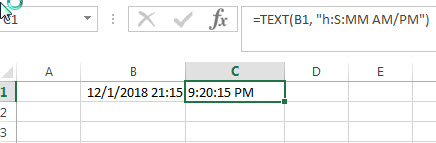For more format codes in excel formatting, you can refer to the below table:

 Format Code Description Examples 0 only display digits in its place #.00 – Forces the function to display two decimal places =Text(34.234,”\$##.00″) result: \$34.23 # Display the placeholder =Text(4.527,”#.##) result: 4.53 . the position of Decimal Point =Text(342.2,”0.00″) result: 342.20 d Day of the month or day of week d- one or two digit number (1-31) dd- two digit number (01-31) ddd-abbreviated day of week (Mon to Sun) dddd-full name of day of week(Monday to Sunnday) =Text(TODAY(),”DDDD”) result: Monday m The Month of the Year m- one or two digit number mm-two digit number mmm-abbreviated month(Jan to Dec) mmmm-full name of month(January to December)) =Text(TODAY(),”MM/DD/YY”) result:11/06/17 y year yy-two digit representation of year(e.g.01,17) yyyy-four digit representation of year(e.g. 2001,2017) =Text(TODAY(),”MM/DD/YY”) result:11/06/17 h Hour h-one or two digit number (e.g. 1,23) hh-two digit number (e.g. 01,23) =Text(14:16,”hh:mm”) result: 14:16 m Minute m-one or two digit representation (e.g. 1,59) mm-two digit representation (e.g. 01,59) =Text(14:16,”hh:mm”) result: 14:16 s Second s-one or two digit representation (e.g. 1,59) ss=two digit representation (e.g. 01,59)

### Related Formulas

• Excel Convert numbers to Text
The Text function will accept a numeric value as the first argument, then based on the format code in the second argument to convert the number to text. You can convert all the standard number formats such as: dates, times, currency to Text string in excel.…
• Convert date to month name with Text Function
If you want to convert the date to a month name, you can use the TEXT function with a specified format code like “mmm”in excel.  You can try to run the following TEXT formula:=TEXT(B1,”mmm”)

### Related Functions

• Excel Text function
The Excel TEXT function converts a numeric value into text string with a specified format. The TEXT function is a build-in function in Microsoft Excel and it is categorized as a Text Function. The syntax of the TEXT function is as below: = TEXT (value, Format code)…